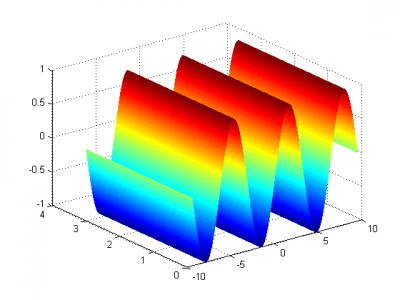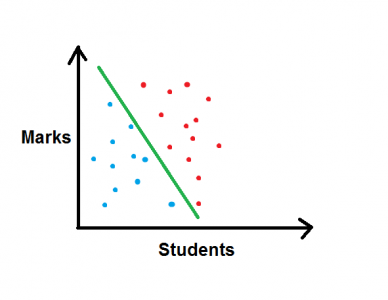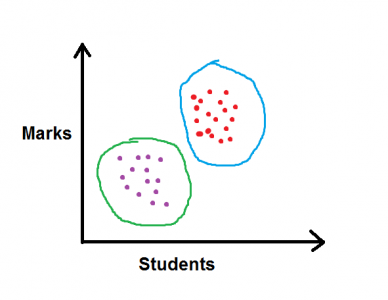| Contact us at durofy@live.com

Machine Learning is a concept which is widely used in today’s world. In a world where no company handles small data, the very fundamentals of Machine Learning (ML) and Big Data become imperative to comprehend. The notion of machine learning is itself very intuitive and helps us further understand the values of Artificial Intelligence (AI) and implement these concepts to construct machines that could further help mankind. So, let us go into the details of ML, as a subject, to understand its applications, contents and visions.A major portion of machine learning is concerned with graphing solutions.

## What is Machine Learning?

The conceptual definition of Machine Learning follows from the general behavior of computer programs. If at any moment, the computer program is supposed to have a certain performance tracker, learning measure and experience with respect to some task, the performance tracker is bound to improve with more exposure to experience. This is analogous to the formal definition set by Tom Mitchell.

To illustrate this stand with an example, we may consider a program as a game of chess, where the task is playing a turn, performance tracker being the win-loss result and the experience being the number of games played.

## Types of Machine Learning

Strictly, there are 2 types of learning algorithms:

a) Supervised learning

b) Unsupervised learning

Supervised Learning

This sort of learning gives us an intuition before hand with respect to a data set we are supposed to analyze. More than an intuition, the supervised learning scheme helps us to predict certain trends in the data sets and helps us to classify and organize such types of data effectively. Let us consider an instance to effectively explain the above.

Assume that you are trying to buy a bottle of roughly 2 L.You, hence have a data set of the prices and sizes of a list of bottles, and you wish to predict some sort of price for the 2L size. We may safely go for a linear plot and try connecting all data points with a straight line and get our required answer. However, the more appropriate approach would include trying to ‘fit’ the data into some other curve, which could best possibly describe the size-cost relationship. This particular problem is known as a regression problem, as we try to predict the result from a continuous input.

Let us take the other case. In a class of say 50 children, some unofficial teacher wants to administer the pass/failure rate of students, and wishes to map the marks into the computer, and wants to get the pass mark. Naturally, those below the pass mark would be failed, and those above would be passed. This kind of discrete analysis of data is described as a classification problem, because the algorithm will separate the pass and the failed students. Mapping the marks on the computer and finding the pass mark is the main objective in this problem.Unsupervised Learning

In unsupervised learning, we do not know whether our data set has all the answers. It is our objective to get the interpretation from the data. For example, if we take the same example as above, and the marks of the students are given, but the fact as to who is passed or failed is not given. Thus, when we plot the marks of the students, we will get clusters of students in either phase. This problem is all about clustering and grouping similar/identically related data points into groups.Share.

### About AuthorCurious Blogger, Undergraduate Electrical Engineer at IIT Kharagpur